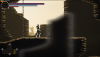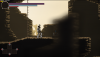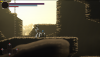# Blur Shader on Transparent Image

#### gats1212

##### Member
Hey guys. I'm using a blur shader to ehh blur layers that are closer to the camera. However this process is not working as intended because there are grey outlines.However I was looking why is this happening and I found that this happens because the blur shader is not intended for transparent images or surfaces. How can I fix this? I tried multiplying the RGB with the alpha.

Code:
``````const int Quality = 16;
const float Distance = 0.1;
const int Directions = 32;

varying vec2 v_vTexcoord;
varying vec4 v_vColour;
const float Pi = 3.14159265359;
const float Tau = Pi*2.0;

void main() {
vec4 Color;
vec4 TempColor;
for(float dir = 0.0;dir<1.0;dir+=1.0/float(Directions)) {
float xvar = (Distance*2.0+Distance)*sin(dir*Tau);
float yvar = (Distance*2.0+Distance)*cos(dir*Tau);

for(float i = 0.0;i < 1.0;i+=1.0/float(Quality)) {
float dis = i*Distance;
TempColor = texture2D(gm_BaseTexture,vec2(v_vTexcoord.x+xvar*dis,v_vTexcoord.y+yvar*dis));
TempColor.rgb *=TempColor.a;
Color += TempColor;
}
}
Color /= float(Quality)*float(Directions)+1.0;
gl_FragColor = Color *v_vColour;
}``````
And this is how I use it:

Code:
``````var xx=camera_get_view_width(view_camera)*2;
var yy=camera_get_view_height(view_camera)*2;
if !surface_exists(surface_front1) {
surface_front1=surface_create(xx,yy);
}
if !surface_exists(surface_front2) {
surface_front2=surface_create(xx,yy);
}

surface_set_target(surface_front1);
draw_clear_alpha(c_white,0);
draw_tilemap(front_tile,-camera_get_view_x(view_camera),-camera_get_view_y(view_camera));
surface_reset_target();

surface_set_target(surface_front2);
draw_clear_alpha(c_white,0);
draw_surface(surface_front1,0,0);

surface_reset_target();
draw_surface_ext(surface_front2,camera_get_view_x(view_camera),camera_get_view_y(view_camera),1,1,0,c_white,1);``````

#### Attachments

• 33.3 KB Views: 6

#### muki

##### Member
in GML, after setting your surface target to surface_front1, try using black when drawing the clear alpha.

draw_clear_alpha(c_black,0);

I do very similar things with my surfaces. drawing tilemaps to surfaces, and applying blur/depth of field to them via shaders.

Last edited:

#### gnysek

##### Member
Transparent images (PNG) still have RGB color set in transparent places (it's just alpha param which changes). Seems that your image is white there. I'm not sure how to set those pixels black+100% transparency, but that seems to be possible.

#### gats1212

##### Member
in GML, after setting your surface target to surface_front1, try using black when drawing the clear alpha.

draw_clear_alpha(c_black,0);

I do very similar things with my surfaces. drawing tilemaps to surfaces, and applying blur/deptch of field to them via shaders.
There was no change between white or black. However the effect SEEMS to be fixed If I use draw_clear_alpha(c_black,0); on the second surface but it's not a real fix. I noticed that, as Gnysek said:
Transparent images (PNG) still have RGB color set in transparent places (it's just alpha param which changes). Seems that your image is white there. I'm not sure how to set those pixels black+100% transparency, but that seems to be possible.
So the shader is actually blending the tileset with black, transparent pixels and I don't want it to happen.Last edited:

#### rytan451

##### Member
You're going to have to modify the shader. I'm not certain, but I think this might work.

Code:
``````const int Quality = 16;
const float Distance = 0.1;
const int Directions = 32;

varying vec2 v_vTexcoord;
varying vec4 v_vColour;
const float Pi = 3.14159265359;
const float Tau = Pi*2.0;

void main() {
vec4 Color;
vec4 TempColor;
float BlurInfluence = 0;
for(float dir = 0.0;dir<1.0;dir+=1.0/float(Directions)) {
float xvar = (Distance*2.0+Distance)*sin(dir*Tau);
float yvar = (Distance*2.0+Distance)*cos(dir*Tau);

for(float i = 0.0;i < 1.0;i+=1.0/float(Quality)) {
float dis = i*Distance;
TempColor = texture2D(gm_BaseTexture,vec2(v_vTexcoord.x+xvar*dis,v_vTexcoord.y+yvar*dis));
TempColor.rgb *=TempColor.a;
Color += TempColor;
BlurInfluence += TempColor.a;
}
}
Color /= float(Quality)*float(Directions)*BlurInfluence+1.0;
gl_FragColor = Color *v_vColour;
}``````

Last edited:

#### gats1212

##### Member
You're going to have to modify the shader. I'm not certain, but I think this might work.

Code:
``````const int Quality = 16;
const float Distance = 0.1;
const int Directions = 32;

varying vec2 v_vTexcoord;
varying vec4 v_vColour;
const float Pi = 3.14159265359;
const float Tau = Pi*2.0;

void main() {
vec4 Color;
vec4 TempColor;
float BlurInfluence = 0;
for(float dir = 0.0;dir<1.0;dir+=1.0/float(Directions)) {
float xvar = (Distance*2.0+Distance)*sin(dir*Tau);
float yvar = (Distance*2.0+Distance)*cos(dir*Tau);

for(float i = 0.0;i < 1.0;i+=1.0/float(Quality)) {
float dis = i*Distance;
TempColor = texture2D(gm_BaseTexture,vec2(v_vTexcoord.x+xvar*dis,v_vTexcoord.y+yvar*dis));
TempColor.rgb *=TempColor.a;
Color += TempColor;
BlurInfluence += TempColor.a;
}
}
Color /= float(Quality)*float(Directions)*BlurInfluence+1.0;
gl_FragColor = Color *v_vColour;
}``````
By doing this the front tiles are not shown.

#### rytan451

##### Member
Oh, dammit. I just noticed the problem in the code. (It never works on the first try).

Code:
``````const int Quality = 16;
const float Distance = 0.1;
const int Directions = 32;

varying vec2 v_vTexcoord;
varying vec4 v_vColour;
const float Pi = 3.14159265359;
const float Tau = Pi*2.0;

void main() {
vec4 Color;
vec4 TempColor;
float BlurInfluence = 0;
for(float dir = 0.0;dir<1.0;dir+=1.0/float(Directions)) {
float xvar = (Distance*2.0+Distance)*sin(dir*Tau);
float yvar = (Distance*2.0+Distance)*cos(dir*Tau);

for(float i = 0.0;i < 1.0;i+=1.0/float(Quality)) {
float dis = i*Distance;
TempColor = texture2D(gm_BaseTexture,vec2(v_vTexcoord.x+xvar*dis,v_vTexcoord.y+yvar*dis));
TempColor.rgb *=TempColor.a;
Color += TempColor;
BlurInfluence += TempColor.a;
}
}
Color /= BlurInfluence+1.0; // Fix here
gl_FragColor = Color *v_vColour;
}``````

#### basementApe

##### Member
Instead of clearing to black or white, you could try to disable drawing the alpha channel when you apply the blur shader. Don't know if it helps in your case but it's gotten rid of unwanted outlines for me before:
GML:
``````surface_set_target(surface_front2);

// disable alpha drawing temporarily
gpu_set_colorwriteenable(true, true, true, false);

draw_surface(surface_front1,0,0);

// re-enable alpha drawing when finished
gpu_set_colorwriteenable(true, true, true, true);``````

•muki

#### gats1212

##### Member
Instead of clearing to black or white, you could try to disable drawing the alpha channel when you apply the blur shader. Don't know if it helps in your case but it's gotten rid of unwanted outlines for me before:
GML:
``````surface_set_target(surface_front2);

// disable alpha drawing temporarily
gpu_set_colorwriteenable(true, true, true, false);

draw_surface(surface_front1,0,0);

// re-enable alpha drawing when finished
gpu_set_colorwriteenable(true, true, true, true);``````
The tileset is not shown if I do this#### gats1212

##### Member
Oh, dammit. I just noticed the problem in the code. (It never works on the first try).

Code:
``````const int Quality = 16;
const float Distance = 0.1;
const int Directions = 32;

varying vec2 v_vTexcoord;
varying vec4 v_vColour;
const float Pi = 3.14159265359;
const float Tau = Pi*2.0;

void main() {
vec4 Color;
vec4 TempColor;
float BlurInfluence = 0;
for(float dir = 0.0;dir<1.0;dir+=1.0/float(Directions)) {
float xvar = (Distance*2.0+Distance)*sin(dir*Tau);
float yvar = (Distance*2.0+Distance)*cos(dir*Tau);

for(float i = 0.0;i < 1.0;i+=1.0/float(Quality)) {
float dis = i*Distance;
TempColor = texture2D(gm_BaseTexture,vec2(v_vTexcoord.x+xvar*dis,v_vTexcoord.y+yvar*dis));
TempColor.rgb *=TempColor.a;
Color += TempColor;
BlurInfluence += TempColor.a;
}
}
Color /= BlurInfluence+1.0; // Fix here
gl_FragColor = Color *v_vColour;
}``````#### muki

##### Member
I might be wrong, but I'm wondering if you even need draw_clear_alpha(c_white,0)
This is how I apply blur to my tilemap surfaces. I don't even draw the clear alpha. Buttery-smooth blur.

GML:
``````//draw background
surface_set_target(surface_canvas_1);
draw_tilemap(layer_background_tilemap,(0-cam_x), (0-cam_y)+8);
draw_tilemap(layer_back_tilemap, 96-cam_x, 64-cam_y);
//draw_sprite_ext(fog, 0, 0, 0, 3, 3, 0, c_white, fog_density);                //fog
surface_reset_target();

surface_set_target(surface_canvas_2);
draw_surface_ext(surface_canvas_1, 0, 0, 0.75, 0.75, 0, c_white, 1);        //0.85
surface_reset_target();``````
I'd share my blur shader as well, but I believe it's this one from the marketplace, so it wouldn't be proper for me to share it. So this may not be helpful. I just can't shake the feeling that this can be fixed outside of the shader.

#### gats1212

##### Member
I might be wrong, but I'm wondering if you even need draw_clear_alpha(c_white,0)
This is how I apply blur to my tilemap surfaces. I don't even draw the clear alpha. Buttery-smooth blur.

GML:
``````//draw background
surface_set_target(surface_canvas_1);
draw_tilemap(layer_background_tilemap,(0-cam_x), (0-cam_y)+8);
draw_tilemap(layer_back_tilemap, 96-cam_x, 64-cam_y);
//draw_sprite_ext(fog, 0, 0, 0, 3, 3, 0, c_white, fog_density);                //fog
surface_reset_target();

I use draw_clear_alpha to clear the surface before the tile is drawn again. If I don't do this, the screen will look like when you win ms solitaire or when you're out of bounds in a 3D game. I'm actually curious why you don't need it, also the blur from the marketplace is not avaliable